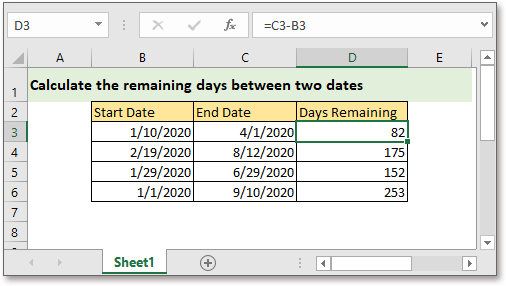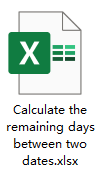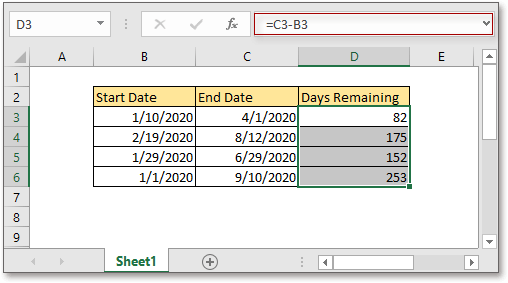## Excel Formula: Calculate days remaining between two datesHere, this tutorial provides a formula to quickly calculate the left days between two dates.Generic formula:

 End_date-Start_date

Arguments

 Start_date: the start date in the date range. End_date: the end date in date range.

Return value

The formula returns the remaining days in a number format.

How this formula work

Supposing calculate the left days between dates in B3 and C3, please use below formula:

 =C3-B3

Press Enter key to get the first result, then drag fill handle to get all remaining days between each date range.